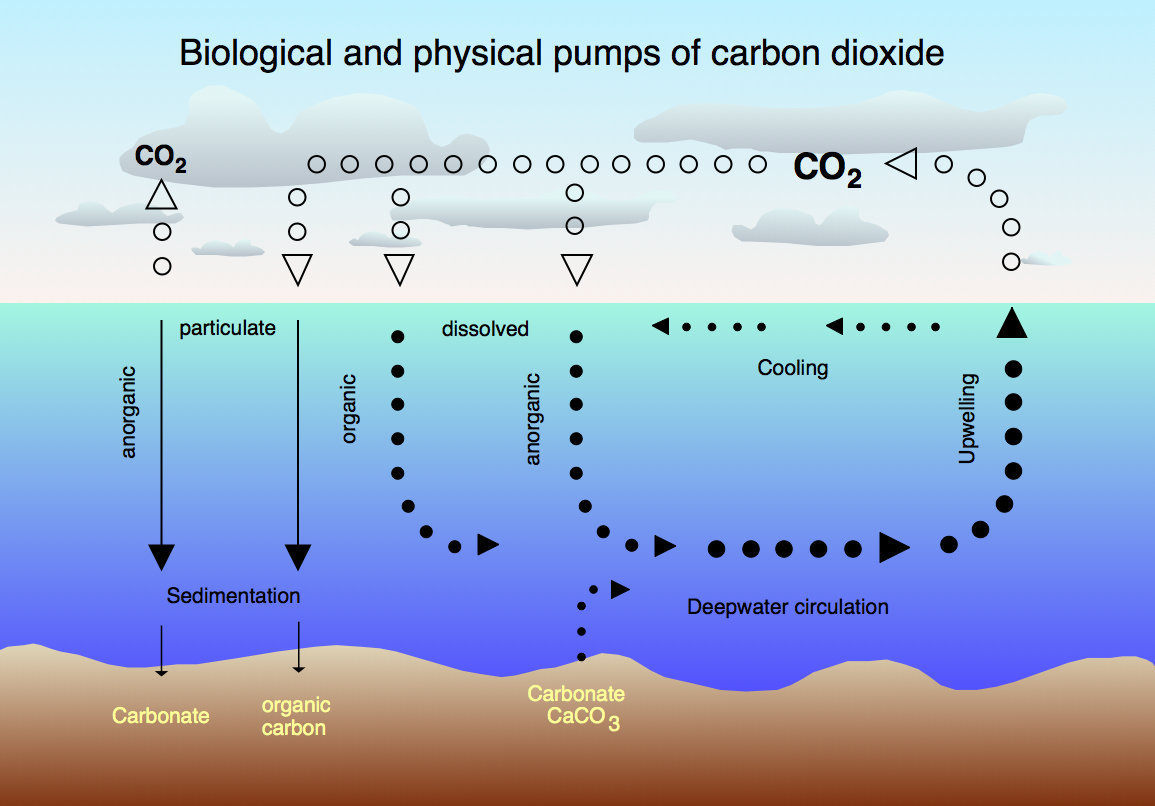# Dependence of the pH on the added carbon dioxide amount#

Written by Svetlana Kyas (ETH Zurich) on Mar 31th, 2022

Attention

Always make sure you are using the latest version of Reaktoro. Otherwise, some new features documented on this website will not work on your machine and you may receive unintuitive errors. Follow these update instructions to get the latest version of Reaktoro!

This tutorial demonstrates the modeling of the pH dependence in the seawater on the CO2(g) amount added, which causes the so-called ocean acidification. This phenomenon remains one of the main problems of human activities, namely the burning of fossil fuels. As carbon dioxide in the atmosphere increases, so does the amount of carbon dioxide absorbed by the ocean. This leads to a series of chemical reactions in seawater that negatively impact the ocean and submerged species. In particular, this leads to decreased production of shells of bivalves and other aquatic organisms with calcium carbonate shells, as well as some other physiological challenges for marine organisms.The CO2 cycle between the atmosphere and the ocean, Source: wikipedia.org

We start by defining the chemical system containing aqueous and gaseous phases.

from reaktoro import *
import pandas as pd

db = SupcrtDatabase("supcrtbl")

# Create an aqueous phase automatically selecting all species with provided elements
aqueousphase = AqueousPhase(speciate("H O C Ca Mg K Cl Na S N"))
aqueousphase.set(chain(
ActivityModelHKF(),
ActivityModelDrummond("CO2"),
))

# Create a gaseous phase
gaseousphase = GaseousPhase("CO2(g)")
gaseousphase.set(ActivityModelPengRobinsonPhreeqc())

# Create the chemical system
system = ChemicalSystem(db, aqueousphase, gaseousphase)

# Create the equilibrium solver
solver = EquilibriumSolver(system)


Next, we initialize the chemical state corresponding to the seawater content, equilibrate it, and evaluate the pH level obtained after equilibration:

state = ChemicalState(system)
state.setTemperature(25, "celsius")
state.setPressure(1.0, "bar")

solver.solve(state)

aprops = AqueousProps(state)
print("pH of seawater = ", float(aprops.pH()))

pH of seawater =  7.699061704247914


Finally, we define the auxiliary variables with the initial values of the CO2 amount and its increment. We run the loop with nsteps steps adding CO2 into seawater and re-equilibrate it again. We collect the amount of added carbon dioxide and the corresponding pH value in pandas.DataFrame.

co2_0 = 0.0
co2_delta = 0.1
nsteps = 50

df = pd.DataFrame(columns=["amountCO2", "pH"])
df.loc[len(df)] = [co2_0, float(aprops.pH())]

for i in range(nsteps):

# Add more CO2 to the problem

# Equilibrate state with updated problem
solver.solve(state)

# Update aqueous properties
aprops.update(state)

# Update CO2 amount
co2_0 += co2_delta

# Append new calculated value to the dataframe
df.loc[len(df)] = [co2_0, float(aprops.pH())]


We plot below pH as a function of the added amount of CO2 into the seawater.

from reaktplot import *

fig = Figure()
fig.title("PH DEPENDENCE ON AMOUNT OF ADDED CO2 TO THE SEAWATER")
fig.xaxisTitle('CO2 [mmol]')
fig.yaxisTitle('pH')
fig.drawLineWithMarkers(df["amountCO2"], df["pH"], name="")

fig.show()